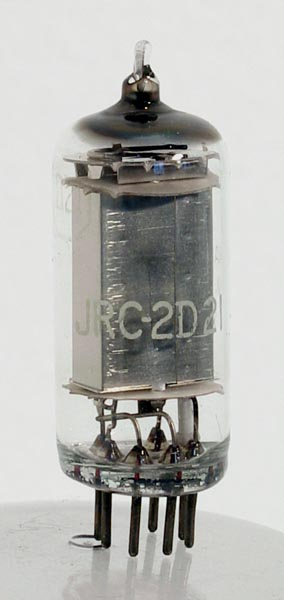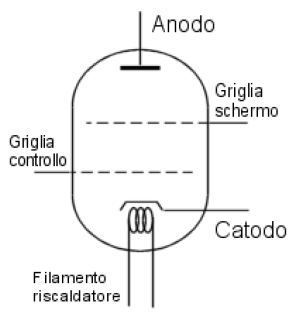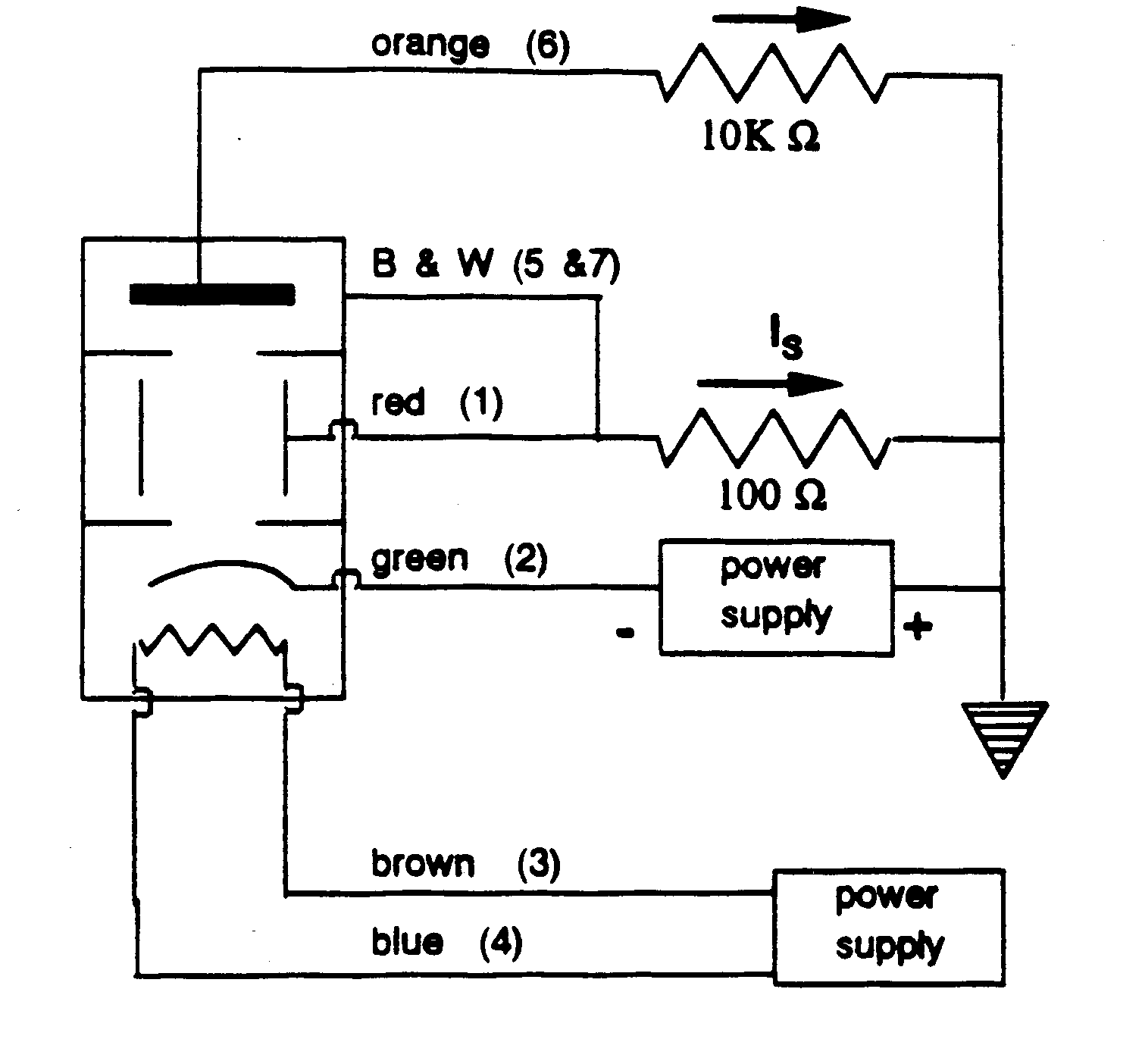Home / English Posts / Ramsauer-Townsend Effect

# Ramsauer-Townsend EffectIn this post we describe a particular characteristic curve of a vacuum tube that allows to estimate the size of an atom of a noble gas.

The Ramsauer – Townsend effect consists in the reduction of the cross section when electrons with around 1 eV energy collide atoms of inert gases : argon Ar , krypton Kr , neon Ne. This reduction can not be explained in terms of classical mechanics as it is connected with the wave nature of the electrons : furthermore, the effect can be explained with a simple one-dimensional model which, although with very simplified assumptions, provides an immediate interpretation and some quantitative data, at least in the orders of magnitude.

The purpose of this experiment is precisely to show how, also on the basis of a one-dimensional model, it is possible to arrive at the estimate of the size of an atom of an inert gas. The effect owes its name to the two researchers, Carl Ramsauer and John Sealy Townsend who discovered it independently of each other, at the beginning of 1920. They observed that for relatively little energy electrons, the probability of collision with noble gas atoms have a minimum value for a given value of kinetic energy. For example for xenon is about 1 eV , after which tends to rise .

An explanation of this effect, related to the properties of the electron wave, can only obtained in quantum mechanics. Assimilating the atom to a simple potential well, it is possible to correlate the energy value at which is obtained the minimum collision likelyhood to the size of the scattering atom.

The experiment here described uses a thermionic tube in which the filler gas (xenon) is used as current limiter, scattering the electrons that contribute to the formation of the anode current – which presents the maximum in correspondence to the  electronic energies which minimize the likelyhood of collision with the gas atoms, causing, in fact, the Ramsauer – Townsend effect.

### Potential Well Scattering

A first estimate of the experimental result is obtained by considering the atom as a one-dimensional potential well. This model allows, partially, to explain the Ramsauer effect.
Let’s start by taking a particle characterized by an energy E. We are also in the presence of a potential well (suppose that the external potential is V = 0 and that the hole has a depth V0 and a length L) :The red arrows indicate the phenomena of reflections that may occur in correspondence of the two “walls” of the “hole”. For the potential well we can easily solve the Schrödinger equation obtaining the following equations representing the particle in the three regions :

At this point we have to link the solutions of the three regions by deriving the various solutions. The fittings are performed for x = 0 and x = L.With the wave functions we can calculate the coefficient of the particle transmission. In the figure on the left we show the trend of the transmission coefficient T as a function of the ratio E/V0 obtained from the equations. Note how there is a situation of resonance for some values of E/V0 wherein T reaches 100%.

The result is interesting : despite it do not represent well the trend that is experimentally observed, it is clear that the T parameter is not monotonous, as expected from classical theory. It is also evident a possible resonance phenomenon, in which the ratio reaches a value equal to 1.

The situation T = 1 is obtained for certain values of k2, for which the potential well is completely transparent to the incoming particle.
Such behavior could correspond to Ramsauer-Townsend effect. By connecting this model to a noble gas atom, we can consider the atom as a potential well of length L = 2R, which corresponds to a minimum of the cross section for a given electron kinetic energy.
Putting R approximately equal to 1 Angstroms, we have that the kinetic energy is about 10 eV. Unfortunately the experimental tests indicate a value for the kinetic energy roughly close to 1 eV, however, the order of magnitude corresponds. To get a better agreement is necessary to adopt more sophisticated models, such as the three-dimensional potential well.

### Experimental SetupThe experiment is essentially identical to what is suggested by Kukolich. In particular, this experiment uses a tetrode tube filled with gas at low pressure xenon (Thyratron). The figure on the left shows the 2D21 model used in this experimental setup. While the right figure shows the simplified diagram of a tetrode, it is a thermionic valve which consists essentially of four elements (in addition to the filament) : anode, cathode, control grid and shield grid, in addition to the terminals for the filament heater cathode.

The advantage of this tube is that the grid is created by a metal shield that envelops the entire central part of the tube, so that it can capture most of the scattered electrons, without damaging the anode with too much high current.

This particular design of the electronic tube is an advantage : in fact the scattered electrons compared to those that have passed free will lead us to understand the transmission ratio and then identify the corresponding minimum of the cross section.

To make the experiment the circuit shown below has been made :The numbers next to the links correspond to the  tube socket pins, as shown in the diagram opposite. The pins 3 and 4 connect the filament and must be powered at a voltage of 3-5 V (although in our measurements we raised this voltage up to 10 V). The cathode is powered at a voltage, negative with respect to the anode, variable from 0 to 5 V. The 100Ω  resistance collects the grid (control and shield grids) current , while the 10 kΩ resistance collects the anode current.

The electronic tube is mounted on a container that houses inside the few components and the connections to the connectors mounted on the sides of the box. The figure below shows the equipment opened.The anode current and the grid current are quantified by measuring respectively the anode voltage and the grid voltage. The experiment is carried out by varying the cathode voltage in small steps and measuring simultaneously the anode voltage and the grid voltage. The measurements were made using a multimeter and a signal generator, as shown in the picture below.The equipment could be improved and automated using a component such as Theremino connected to an ADC24 (analog – digital converter 24bit). In this way the variable cathode voltage may be generated directly from Theremino, while the anode  and grid voltages may be acquired by the ADC converter with high precision :The signals may be acquired by software through the Theremino system data logger :### Results and Data Analysis

The measurements have been carried out by feeding the filament with a voltage of 10 V to 0,75 A. The cathode voltage was varied from 0 to 4.0 V in steps of 0.1 V. Both the anode voltage and the grid voltage have been measured. The following diagrams shows the results.The first graph shows the grid voltage that has, as one would expect, an increasing trend with increasing cathode voltage. The second graph shows the anode voltage that instead presents a relative maximum, which means that this is an unexpected “transparency” to the low energy electrons.

The electrons emitted from the cathode by thermionic effect are accelerated by the cathode voltage toward the anode, however, they are scattered by the molecules of xenon gas; the control grid and, above all, the shield grid collect the scattered electrons that are in the majority, for this reason the shield voltage is much greater than the anode voltage and it increases as the cathode voltage .
The anode is however reached only by a part of the emitted electrons, for this reason, the anode voltage is rather low, also it shows a maximum around the voltage of 1 V, at this value the scattering of xenon gas is minimum.

In our case the maximum is in correspondence with the cathode voltage :

Vmax=1.25 V

In good agreement to the theoretical value.

### Reference

Among the many works on the effect RT, we refer particularly to the following report
Effetto Ramsauer – Townsend in un Thyratron (Xenon) – (Monaci, Fusco)
from which we were inspired for this project.

If you liked this post you can share it on the “social” Facebook, Twitter or LinkedIn with the buttons below. This way you can help us! Thank you !

#### Donation

If you like this site and if you want to contribute to the development of the activities you can make a donation, thank you !## Alpha Detector with BPX61 Photodiode

Abstract : in this post we aim to examine the possibility of using the BPX61 …